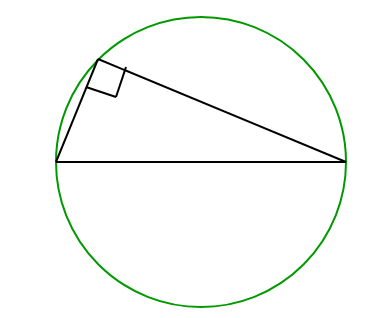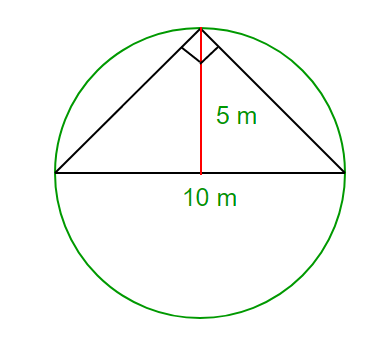Related Articles

# Puzzle 67 | Fit Triangle

• Difficulty Level : Hard
• Last Updated : 21 Jul, 2021

Sameer asks his friend Kartik a question to test his intelligence.

Sameer – You have been given a right-angled triangle ABC, right-angled at B. The hypotenuse length is 10m and the altitude to the hypotenuse from B is 6m.

Kartik – Go on.

Sameer – Tell me the area of the triangle ABC.

Kartik – 30 sq. Meters.

Sameer – I knew you would say this, but you’re wrong my friend. Try some geometry.

Answer: Such a triangle can never exist

Solution: At first it would seem that 30 sq. Meters is the correct answer, using the formula

0.5*Base*Height
=0.5*10*6
=30

A triangle with given dimensions can never exist. The maximum altitude to the hypotenuse of a triangle can be half of the length of the hypotenuse. (See pic)So the maximum altitude can be half of the diameter, which is 10m in this case. Hence the maximum altitude to the hypotenuse can be half of 10m, i.e 5m only.